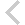FICO Xpress Optimization Examples Repository
 FICO Optimization Community FICO Xpress Optimization HomeLoad and solve a simple 2x2 linear program

Description

We load and solve a 2x2 linear program

Further explanation of this example: Xpress R Reference Manual

Source Files
By clicking on a file name, a preview is opened at the bottom of this page.

first_lp_problem.R

#####################################
# This file is part of the          #
# Xpress-R interface examples       #
#                                   #
#   (c) 2022 Fair Isaac Corporation #
#####################################
#' ---
#' title: "First LP Problem"
#' ---
#'
#' For starters, we solve the following 2-variable model from R using the Xpress-R interface
#'
#' #' \begin{align} #' & \min & x_1 + x_2 \\ #' & & 5 x_1 + x_2 & \geq 7 \\ #' & & x_1 + 4 x_2 & \geq 9 \\ #' & & x_1, x_2 & \geq 0 #' \end{align} #'
#'
## ----first LP problem---------------------------------------------------------
suppressMessages(library(xpress))
library(magrittr)

# create a list object to hold all input
problemdata <- list()

# objective coefficients
problemdata$objcoef <- c(1,1) # row coefficients as a single matrix object problemdata$A <- matrix(c(5,1,1,4), nrow=2, byrow=TRUE)

# right-hand side
problemdata$rhs <- c(7,9) # row sense problemdata$rowtype <- c("G", "G")

# lower bounds (automatically 0 if not present)
problemdata$lb <- c(0,0) # upper bounds(automatically inferred if not present) problemdata$ub <- c(Inf,Inf)

# names for writing to MPS/LP files
problemdata$colname <- c("x_1", "x_2") # Problem Name displayed when the solver solves the problem. problemdata$probname <- "FirstExample"

#'
#' We now load everything into a new XPRSprob 'p'. xprs_loadproblemdata
#' can be used to load all problem data at once into an existing or new
#' problem object. In this case, we have no existing XPRSprob object.
#' xprs_loadproblemdata creates one for us. For convenience and the use
#' inside pipes, xprs_loadproblemdata returns the prob pointer.
#'
## ----Load Problem Data into new Problem---------------------------------------
# You may also use the equivalent
# p <- createprob()

#'
#' The newly created XPRSprob object supports the print and summary
#' statements. Let's get an overview of the optimization problem loaded into p
#'
## ----Printing the Problem-----------------------------------------------------
print(p)

#'
#' We use xprs_optimize to solve the model. This again returns 'p', which can be summarized using the
#' base function summary
## ----Solving and Summarizing the Solution Process-----------------------------
summary(xprs_optimize(p))

#'
#' It may be useful for further processing to convert the solution into a data frame
## ----Convert Solution into a Data Frame---------------------------------------
print(data.frame(Variable=problemdata\$colname, Value=xprs_getsolution(p)))

#'
#' # Using a pipe workflow
#'
#' If you are familiar with pipes in R, you can use the following, one-line
#' workflow to obtain the solution as above. The pipe operator %>% requires
#' loading the magrittr package.
#'
#' The following call creates a new problem object into
#' which the problem data is loaded. Then, it passes the newly created problem
#' object into xprs_optimize, and xprs_getsolution.
#'
#' The result of this call is a
#' solution vector, not the problem!
#'
## ----Using Pipe Workflows-----------------------------------------------------